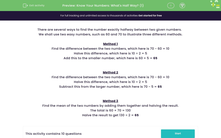# Know Your Numbers: What's Half Way? (1)

In this worksheet, students find the number that is exactly half way between the two given numbers.Key stage:  KS 2

Curriculum topic:   Number: Number and Place Value

Curriculum subtopic:   Solve Number Problems to 1 000 000

Difficulty level:#### Worksheet Overview

There are several ways to find the number exactly halfway between two given numbers.

We shall use two easy numbers, such as 60 and 70 to illustrate three different methods.

Method 1

Find the difference between the two numbers, which here is 70 - 60 = 10

Halve this difference, which here is 10 ÷ 2 = 5

Add this to the smaller number, which here is 60 + 5 = 65

Method 2

Find the difference between the two numbers, which here is 70 - 60 = 10

Halve this difference, which here is 10 ÷ 2 = 5

Subtract this from the larger number, which here is 70 - 5 = 65

Method 3

Find the mean of the two numbers by adding them together and halving the result.

The total is 60 + 70 = 130

Halve the result to get 130 ÷ 2 = 65

### What is EdPlace?

We're your National Curriculum aligned online education content provider helping each child succeed in English, maths and science from year 1 to GCSE. With an EdPlace account you’ll be able to track and measure progress, helping each child achieve their best. We build confidence and attainment by personalising each child’s learning at a level that suits them.

Get started